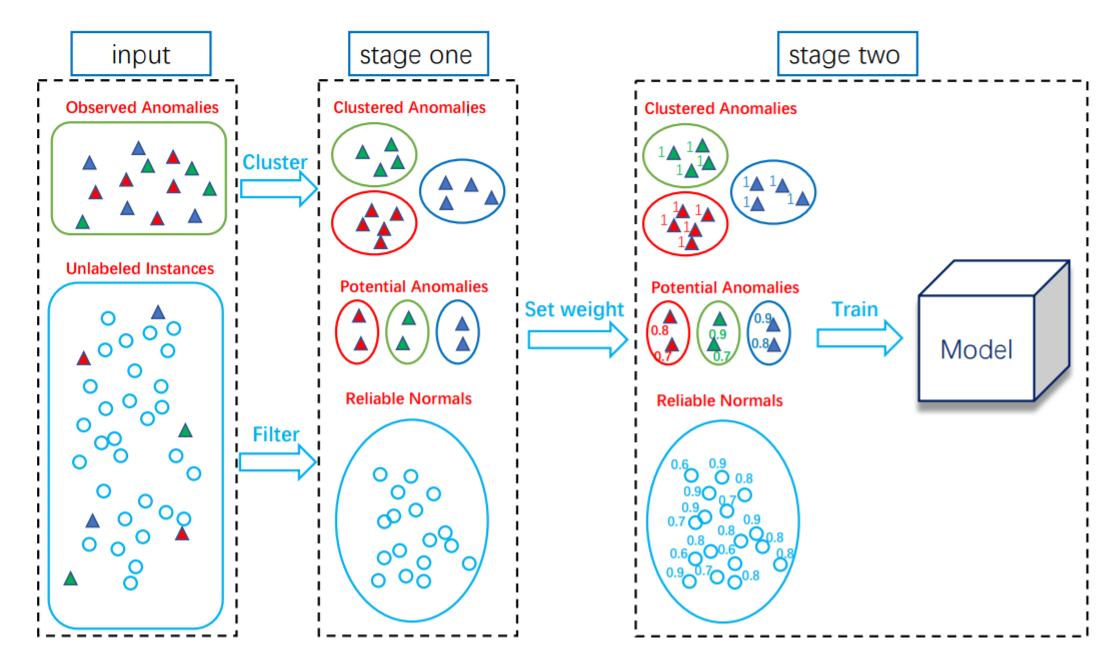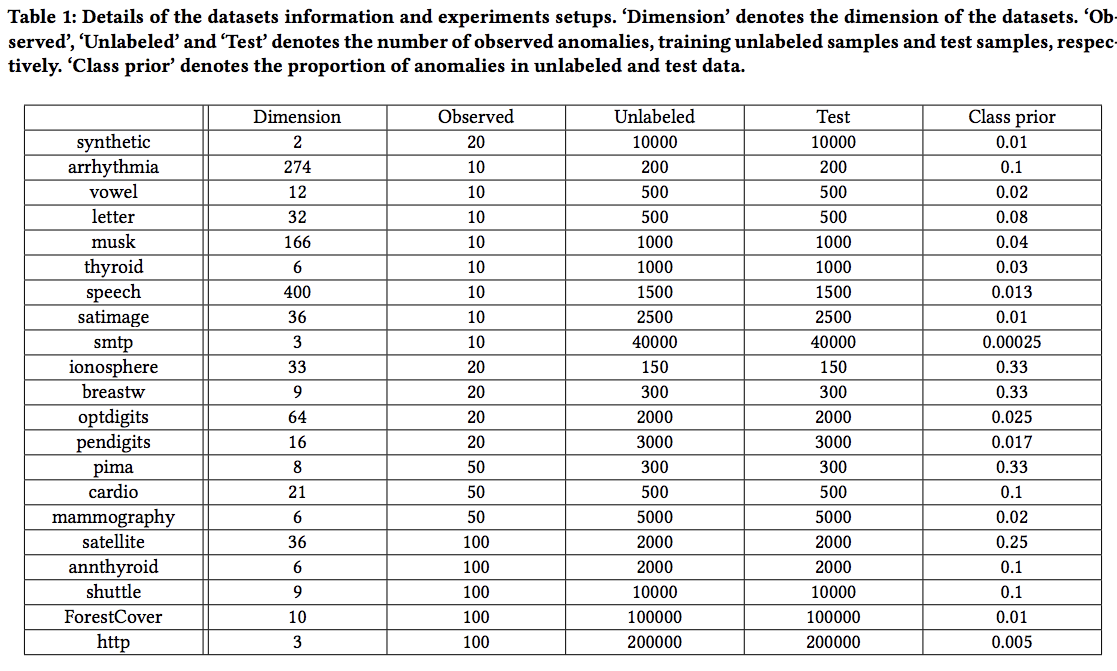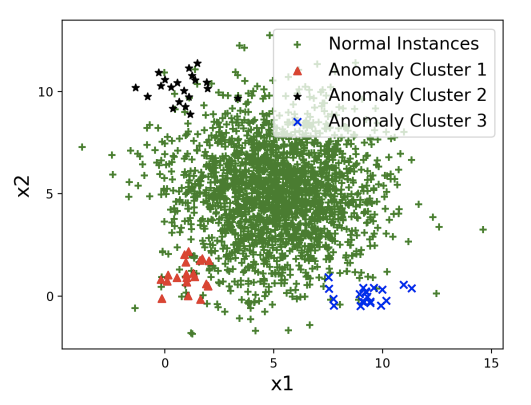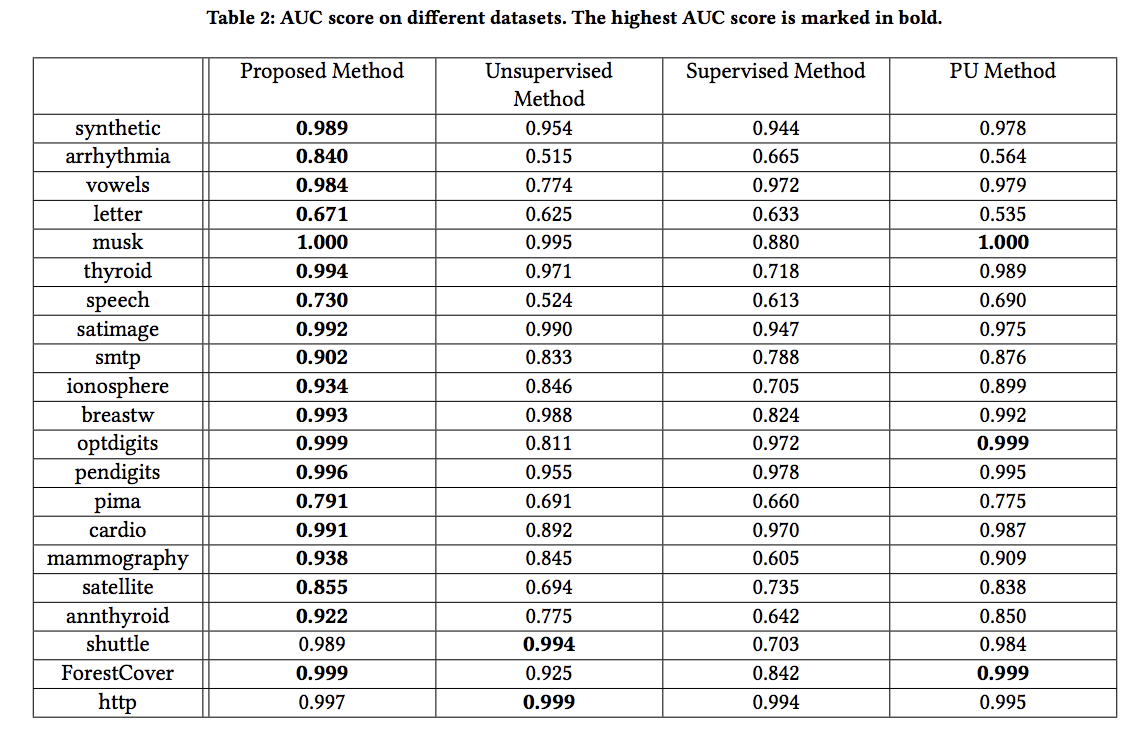# 关键词

## 1. 引言

• step1： 我们认为异常数据不应简单地看成一个中心，假设异常属于k个不同的中心，首先将异常聚类为k个中心。然后根据孤立度与最近异常类中心的相似性，从未标记的样本中选取潜在异常和可靠正样本。
• step2：依据所附标签的置信度，对各个点进行赋权，然后，利用原始异常样本和所选的样本，建立加权的多分类模型，将异常与正常样本区分开来。本文在不同数据集和实际应用任务上的实验中证明了该方法的有效性。
本文的其余部分组织如下。在第2节中，我们回顾了相关工作。在第3节中，我们陈述了问题的设定，并提出了所提出的方法。在第4节中，我们报告了在不同数据集上的实验结果。在第5节中，我们将该方法应用于恶意URL检测问题，并验证了该方法的有效性。最后，我们在第六部分对本文进行了总结。

## 2. 具体工作

PU算法是半监督学习里面的一个特例，它主要用来解决当只有正样本和未标注的样本的数据的时候。过去几年，里，非常多的方法被提出来用以解决这种问题，大致来说，可以分为三类，两步法尝试从未标记的数据中识别出一些可靠的负样本，然后应用传统的监督学习或半监督学习技术。代价敏感学习技术用于不相等误分类代价的二元分类，也可以很容易地用于处理这个问题。针对这一问题，还提出了凸方法。注意，如果我们在这里将异常视为正样本，那么PU学习与部分观测异常的异常检测（ADOA）有些相似。然而,最显著的区别是,正样本在PU学习彼此相似，因此我们可以只找到一个中心，在异常检测，异常总是多样化,他们很少能将其只看做一类，使标准PU学习技术不适合处理异常检测的任务。

## 3. 部分异常观测的异常检测

### 3.1 问题描述和符号标记

$X = R^d$描述的是数据属于实数空间， $y=\{-1,+1\}$ 描述的是标签的范围，其中y=+1指的是异常，-1位正常样本，假设有m个训练样本 $D=\{(x_1,y_1),...(x_l,y_l),...(x_m,y_m)\}$，其中 $x_i \in X$，前 $l$个样本的标记是异常，另外 $m-l$个样本为未标记样本，尽管前 $l$个样本已经标记为异常，但是他们直接还是不同的。目标是建立模型： $f:X \rightarrow Y$，从而进一步利用该方法对未来数据进行各种异常与正常的识别。

### 3.2 本文的方法

ADOA 安装如下两步来进行：

• step1：对于已经标注异常的数据，我们将其分为不同的类，所以这样不同的类里面他们的特征就比较近似，对于未标注的数据，我们的目标是充分挖掘他的价值。所以我们尝试将其分为潜在的异常数据和可靠的正常数据，区分的条件就是isolation score和他们的similarity score。直接原因是这样：一方面，潜在的异常应该和正常样本不一样，另一方法，他们应该可以已观测到的异常近似。
• step2：建立一个加权多分类模型，对于已经标注的异常，赋权为1，对于过滤出来的数据，其权重依据它所带的标签的置信度。

stage1：我们将观测的异常 $D^l$分为k个中心 $C=\{ C_1,C_2,...,C_k \}$，我们可以使用不同的聚类方法，这里只是简单的使用了k-means方法（需要先标准化数据），特别的，两个样本之间的距离直接使用了欧式距离
$dist(x_i,x_{i'})=\sum _{i=1}^n (x_{ij}-x_{i'j})^2$
Isolation score 的计算：孤立的概念第一次被提出来。他们展示随机树林可以用来分离样本，森林里的每棵决策树都使用随机的特征来分割子样本，并不断的分裂节点，知道无法再分割样本。由于异常样本很少，他们总是很贴近于树的根节点，且正常的样本会处于树中比较深的节点。为了计算isolation score，每个样本被送到一颗树的叶子节点上，则可以获得节点的深度，然后计算样本的平均深度。通过计算样本在树中的平均深度，可以认为是样本为异常的概率 $IS(x)$，使 $h(x)$表示样本x的平均深度， $E(h(x))$表示样本在所有孤立树中的平均深度，假设我们有n个样本，则使
$c(n) = 2H(n) -(2(n-1)/n)$

$IS(x) = 2^{-\frac{E(h(x))}{c(n)}}$
$IS(x)$的分数越高（越接近1），样本x就越有可能是异常。
Similarity score 的计算：另一方面，一个样本如果越靠近异常的中心，则它越有可能是潜在的异常，所以我们可以计算样本x和他相近的异常中心的相似分 $SS(x)$，其计算公式如下：
$SS(x) = \max_{i=1}^k e^{-(x-u_i)^2}$

Total score 的计算：为了从未标注的样本中区分潜在的异常和正常样本，我们同时考虑Isolation score和Similarity score，总的得分计算：
$TS(x) = \theta IS(x) + (1-\theta)SS(x)$

$\alpha = \frac{1}{l} \sum_{i=1}^l TS(x_i)$

$w(x) = \frac{TS(x)}{max_x{TS(x)}}$

$w(x) = \frac{max_x{TS(x)} - TS(x)}{max_x{TS(x)} -min_x{TS(x)} }$

$\sum_{i} w_il(y_i,f(x_i))+ \lambda R(w)$

## 实验（略）

• 实验的数据集规模• 第一个数据集的异常分布情况• 不同方法的对比效果图0条评论